Function Repository Resource:

# Proportions

Get the proportion of times that each distinct element appears in a list

Contributed by: Richard Hennigan (Wolfram Research)
 ResourceFunction["Proportions"][list] gives an association whose keys are the distinct elements of list, and whose values give the proportion of times those elements appear in list.

## Details and Options

ResourceFunction["Proportions"][list] gives an association whose keys are in the same order as they first occur as elements of list.
ResourceFunction["Proportions"] also works with heads other than List.
When given an Association, ResourceFunction["Proportions"] uses the Values of the association as input.

## Examples

### Basic Examples (4)

Find the proportions of times that each element appears in a list:

 In:=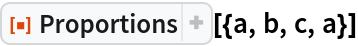Out=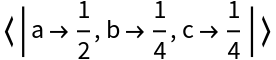Use with an Association:

 In:=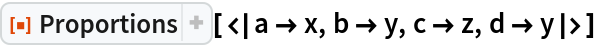Out=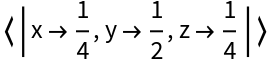See how common each character is in a body of text:

 In:=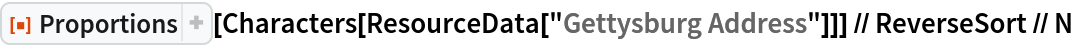Out=Check for common words:

 In:=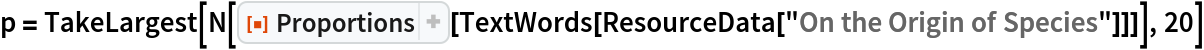Out=In:=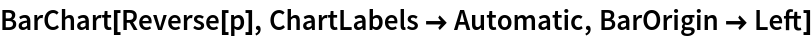Out=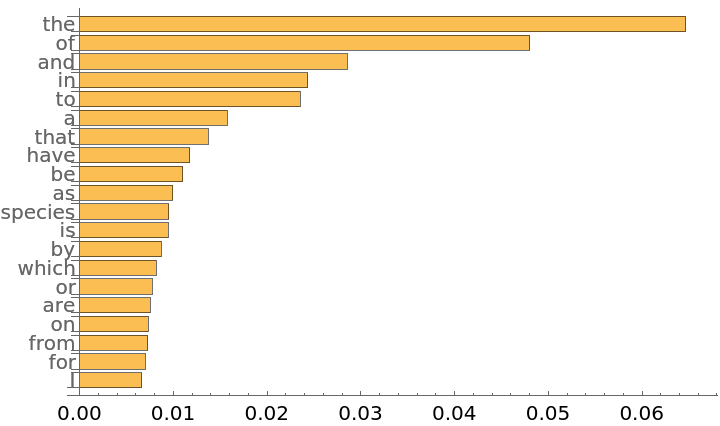Visualize in a WordCloud:

 In:=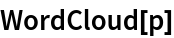Out=### Properties and Relations (1)

Proportions is similar to Counts:

 In:=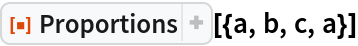Out=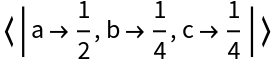In:=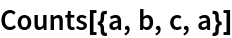Out=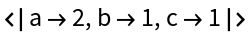In:=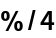Out=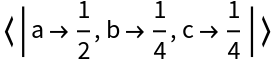## Requirements

Wolfram Language 11.3 (March 2018) or above

## Version History

• 1.0.0 – 28 March 2019